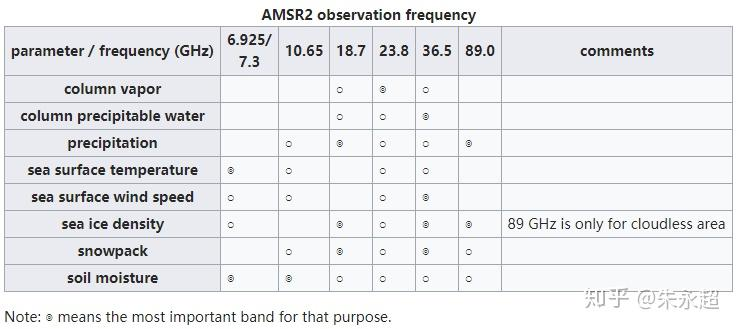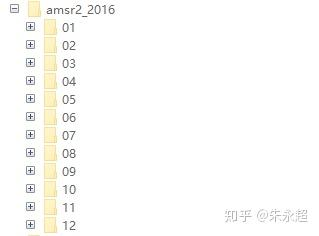# AMSR2土壤水分产品的下载与Matlab读取

## 本文介绍了：

1. AMSR2卫星的背景；

2. 如何在AMSR2官网下载AMSR2数据；

3. 如何在Matlab中读取AMSR2的L3土壤湿度数据。

AMSR2卫星简介-机翻（参见：Global Change Observation MissionAMSR2主要的产品以及相关的观测频段

## 哪里可以下载AMSR2的土壤湿度数据？## 如何利用Matlab读取AMSR2数据？

% 指定你的数据所存放的路径
filedir = 'H:\workfile_Papaer201709\comparison_zidongzhan_VS_fy3_171205\amsr2_2016';
% 获取所有的子文件夹filename = dir(filedir);
% 你数据的顺序（一年中的第多少个）
dateOrder = 0;
% 存放读取后的土壤水分数据（供后续分析使用）
sm_amsr2_2016 = cell(1,365);
% 存放读取后的土壤水分数据的观测时间（供后续分析使用）
date_amsr2_2016 = NaT(1,365);

for imonth=1:12
disp(imonth)

% 当月中的所有土壤湿度文件
filedir_thisM = [filedir ,'\',filename(imonth+2).name];

% 当月的所有数据文件
filenamesA_thisM = dir([filedir_thisM,'\','*D_EQMA_*h5']);
filenamesD_thisM = dir([filedir_thisM,'\','*D_EQMD_*h5']);

fileLength = length(filenamesA_thisM);

if imonth>1
dateOrder = dateOrder+eomday(2016,imonth-1);
end

for ifile = 1:fileLength
disp(ifile)
FILENAME_A = filenamesA_thisM(ifile).name;
FILENAME_D = filenamesD_thisM(ifile).name;

dateOrder2 = dateOrder+ifile;
sm_amsr2_2016{dateOrder2} = datas;

% 获取卫星数据的日期
Idate = strfind(FILENAME_A,'GW1AM2_');
date = FILENAME_A(Idate+7:Idate+14);

date_amsr2_2016(dateOrder2) = datetime(date,'InputFormat','yyyyMMdd');
end
end

save sm_amsr2_2016
save date_amsr2_2016

%   此处显示详细说明

file_id = H5F.open (FILE_NAME, 'H5F_ACC_RDONLY', 'H5P_DEFAULT');

DATAFIELD_NAME = 'Geophysical Data';

data_id1 = H5D.open (file_id, DATAFIELD_NAME);

datas = H5D.read(data_id1,'H5T_NATIVE_DOUBLE', 'H5S_ALL', 'H5S_ALL', 'H5P_DEFAULT');

% Close and release resources. 关闭文件指针
H5D.close (data_id1);
H5F.close (file_id);

%% Replace the fill value with NaN.
datas(datas<-10000) = NaN;

datas = reshape(datas,1440,720);

end

image_combine_amsr2函数的代码：
function [ out_combine ] = image_combine_amsr2( varargin )
%IMAGE_COMBINE 此处显示有关此函数的摘要
%   此处显示详细说明

%   获取输入变量的大小
dim = size(varargin{1});
% 用于放数据
vsm_combine = zeros(dim(1),dim(2));
% 用于计算个数（空值为非覆盖区）
I_combine = zeros(dim(1),dim(2));
% 用于结果数据
out_combine = nan(dim(1),dim(2));

for i = 1:nargin  % 输入变量的个数

% 转换为double类型
varargin{i} = double(varargin{i});

varargin{i}(varargin{i}<-999) = nan;

% 湿度数据
varargin{i} = varargin{i}/1000;
% 当前矩阵中的非空值
I1 = ~isnan(varargin{i});

% 把其中不是空值的部分给矩阵
vsm_combine(I1) = vsm_combine(I1) + varargin{i}(I1);

% 非空格子计数加一
I_combine(I1) =  I_combine(I1)+1;

end

% 输出矩阵
out_combine(~isnan(I_combine)) = vsm_combine(~isnan(I_combine))./ ...
I_combine(~isnan(I_combine));

out_combine = out_combine';
end

https://blog.sciencenet.cn/blog-3367669-1153783.html

## 全部精选博文导读

GMT+8, 2023-2-8 01:47